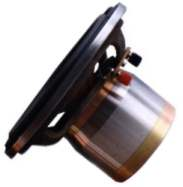[an error occurred while processing this directive]
The REPS R-1 Drive Unit

The Reps 1 represents state of the art in full range drivers. Precice machining with exceptionally tight tolerences and carefully chosen components have produced the finest full range driver ever. A new level of transparency and realism are now obtainable.

The Alcomax 5 magnet, in conjunction with the cobalt alloy pole cap, produces 20,000 Gauss and 100 dB/W at 1 m. This is the strongest type of alnico motor that can be reliably manufactured.

The voice coil is a most geometrically effective design. It is double wound and interleaved around one of the strongest papers available at only 0.075 mm ( 0.003" ) thick.

The cone, which is exceptionally light and rigid, is made of paper and specially treated for humid climates.

This type of design and construction assembled meticulously allows the driver to surpass all other designs.

The massive motor/low mass
cone topology of the
REPS R-1 faithfully
reproduces the
minute details
of original
music
with less
than five watts
of inputS P E C I F I C A T I O N S

 Drive Unit Size: 200 MM Nominal Frequency Response, Box Dependent 40Hz to 20,000Hz +/- 3dB Sensitivity, 1 Watt at One Meter: 99.8 dB Power Handling, Sine Wave: 55 Watts RMS Suggested Amplifier Power: 2 to 45 Watts RMS Distorion at 100 dB SPL: Less than 0.15%

Electrical Specifications

Speaker Parameter Measurement Data (SPM)

Method: Delta Compliance Curve Pair

 Free Air Curve Num = 13 Name = REPS 1 LIGHT FREE-AIR Delta Comp Curve Num = 14 Name = REPS 1 LIGHT BOX 29L

Volume of Test Box = 29.00 Liter = 1.02 cuFt

Electrical/Mechanical Parameters

 Revc (DC VC Res) = 6.4 Ohm Qms (Mech Q) = 5.98 Fo (Res Freq) = 39 Hz Qes (Elec Q) = 0.107 Zo (Zmax at Fo) = 371 Ohm Qts (Total Q) = 0.105 Sd (Piston Area) = 0.0206 sqM Vas (Acous Vol) = 101.29 litr BL (Flux*Length) = 12.1 TM Cms (Compliance) = 1680.8 uM/N no (Ref Effncy) = 5.4 % Mms (Total Mass) = 9.96 Gram SPLo (SPL at 1W) = 99 dB Mmd (Diaphm Mass) = 8.26 Gram

Motor Impedance Parameters

 Levc (Induc at 1kHz) = 0.446 mH Rem (Res at 1KHz) = 2.14 Ohm Levc (Induc at 20kHz) = 0.065 mH Rem (Res at 20kHz) = 9.93 Ohm Krm (Resistance Cons) = 24.077 mOhm Erm (Resis Expont) = 0.52 Kxm (Reactance Cons) = 121.332 mH Exm (React Expont) = 0.36
[an error occurred while processing this directive]